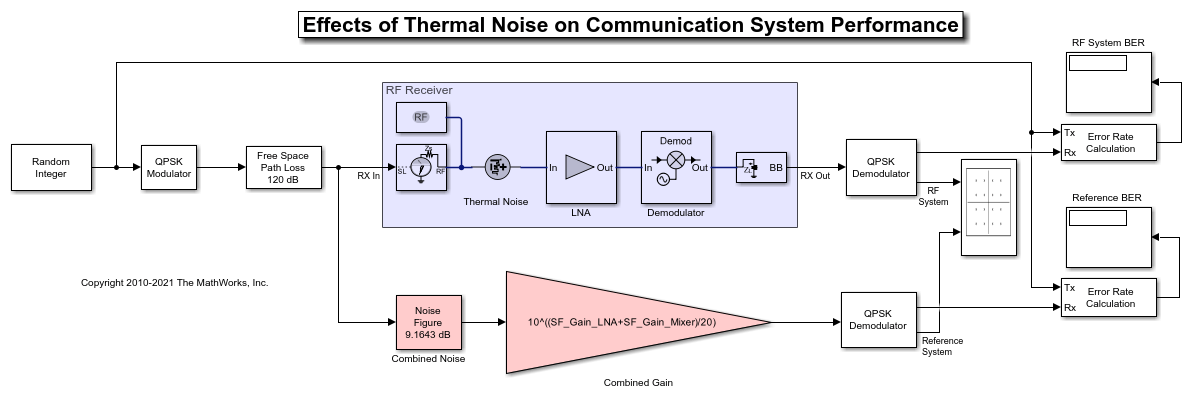Documentation

## Impact of Thermal Noise on Communication System Performance

This example shows how to use the RF Blockset Circuit Envelope library to model the thermal noise of a super-heterodyne RF receiver and measure its effects on the bit error rate (BER) of a communications system. The RF receiver model does not include impedance mismatches or non-linearity. A Communications Toolbox reference model with parameters computed using Friis-equations is used to verify the results.

### RF Receiver System Architecture

The Modulator and Channel subsystems consist of Communications Toolbox blocks that model:

• A QPSK-modulated waveform of random bits

• A raised cosine pulse-shaping filter for spectral limiting

• Free-space path loss

The RF receiver subsystem, shown in the light purple area, consists of:

• An Inport block, which assigns the input complex waveform to the specified RF carrier, in this case equal to the variable SF_RF = 2.1GHz. To ensure that the RF Blockset model has the same available power referred to a 50 Ohm impedance as the reference model, the Source type parameter is set to Power.

• A cascaded RF amplifier and RF demodulator with specified noise figure and gain.

• An Outport block, with the Source type parameter set to Power and center frequency equal to the intermediate frequency given by the absolute difference between the RF center frequency specified in the Inport and Local Oscillator specified in the demodulator. In this case, the intermediate frequency is equal to the variable SF_IF = 500MHz.

• Note the presence of the thermal white noise source at the input of the chain to model the equivalent noise floor introduced by an ideally matched antenna (50Ohm) at 290K.

• Note the presence of an image reject filter at the input of the demodulator. This filter ensures that only signals with frequency around the carrier at 2.1 GHz enter the demodulator. Thanks to the image rejection filter, the thermal noise centered around 2.6GHz (the image frequency is equal to SL_RF+SL_IF) is not down-converted to the intermediate frequency. If you remove the image rejection filter, the noise contribution on the output signal increases above the estimation provided by Friis equations, and thus the BER deteriorates.

• Note that, as the amplifier and the mixer do not specify any non-linearity, the harmonic order of the simulation specified in the configuration block is set to 1.

• Note that all blocks in the RF receiver are ideally matched to 50Ohm.% To understand the effects of impedance mismatches on noise simulation, please see the example `RF Noise Modeling` .

The reference system, shown in red, consists of:

• A Communications Toolbox Receiver Thermal Noise block that adds noise to the signal according to the value calculated by the Friis Equation. You can find the calculation in the in the model pre-load callback function. This block also includes the equivalent thermal noise introduced by the antenna.

• A Gain block that models the combined gain of the RF receiver. Communications Toolbox filters and demodulators process the received signal.

### Circuit Envelope Simulation of the RF Receiver

``` open_system('RFReceiverImpactExample') ```Select Simulation > Run.

Error Rate Calculation blocks compute the BER for the system and reference. To observe the BER as it approaches steady state, increase the total simulation time. For this example, the steady-state bit error rate is approximately 0.17.

### Computing RF Receiver Noise Figure

To model noise in the RF Blockset circuit envelope environment:

* In the Configuration block dialog, select Simulate noise. * Specify the Noise figure (dB) parameter of RF Amplifier and RF Mixer blocks in your system. The following specifications for the RF receiver in this example produce a combined noise figure of 6.3218 dB (as per the Friis Equation): LNA gain of 20 dB, LNA noise figure of 6 dB, and RF mixer noise figure of 15 dB.